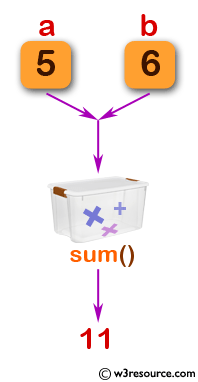﻿ C Program: A simple structure of function - w3resource

# C Exercises: A simple structure of function

## C Function : Exercise-1 with Solution

Write a program in C to show the simple structure of a function.

Pictorial Presentation:Sample Solution:

C Code:

``````#include <stdio.h>

int sum (int, int);//function declaration
int main (void)
{
int total;
printf("\n\n Function : a simple structure of function :\n");
printf("------------------------------------------------\n");
total = sum (5, 6);//function call
printf ("The total is :  %d\n", total);
return 0;
}

int sum (int a, int b) //function definition
{
int s;
s=a+b;
return s; //function returning a value
}
```
```

Sample Output:

``` Function : a simple structure of function :
------------------------------------------------
The total is :  11
```

Explanation:

```int sum(int a, int b) //function definition
{
int s;
s = a + b;
return s; //function returning a value
}
```

The above function ‘sum’ takes two integer arguments, a and b. It computes the sum of a and b and stores the result in a local integer variable s. Finally, it returns the value of s.

Time complexity and space complexity:

The time complexity of the function 'sum' is O(1), as the computation it performs is constant and independent of the input size.

The space complexity of the function 'sum' is also O(1), as it only uses a fixed amount of memory to store the integer variable 's'.

Flowchart:C Programming Code Editor:

What is the difficulty level of this exercise?

Test your Programming skills with w3resource's quiz.

﻿